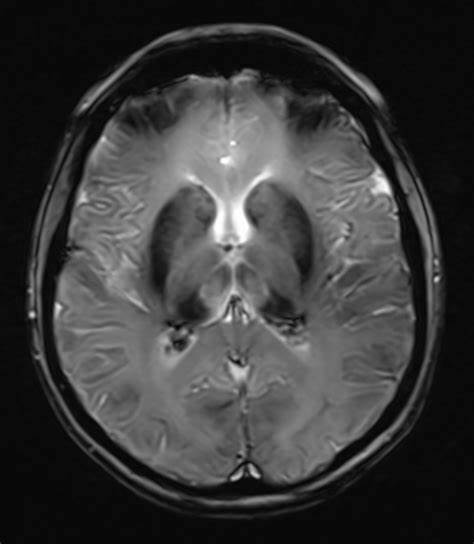# The serum insulin concentration for TC, LDL-C HDL-C, TG, creatinine, CRP, gamma glutamyltransferase (GGT), total calcium, and albumin, and the plasma

diabetes-obesity-2015-10-26

## The serum insulin concentration for TC, LDL-C HDL-C, TG, creatinine, CRP, gamma glutamyltransferase (GGT), total calcium, and albumin, and the plasma

### Blood Sampling & Laboratory Analyses

Blood samples were collected in the early morning (7.00–9.00 a.m.) after an overnight fast (12 h). The serum was assayed for TC, LDL-C HDL-C, TG, creatinine, CRP, gamma glutamyltransferase (GGT), total calcium, and albumin, and the plasma was measured for glucose (Horiba ABX Pentra 400), and TSH (ARCHITECT ci8200, Abbott Diagnostics). The serum insulin concentration was determined using a sandwich ELISA method: Insulin: DRG MedTek, with intra-assay precision of 2.8–4.0%, and inter-assay precision of 2.6–3.6%; BCAAs were assayed using a colorimetric enzyme test (Kit Immundiagnostik AG, Bensheim, Germany for the in vitro determination of total BCAAs as a sum of L-leucine, L-isoleucine and L-valine in serum) with intra-assay precision of 4.2–5.5%, and inter-assay precision of 3.7–8.9%.

• The EDTA blood samples were analyzed for glycated hemoglobin (HbA1c) using a Bio-Rad VARIANT
• II turbo (HPLC). The HOMA-IR value was calculated by dividing the fasting
• insulin concentration (mU/L) and the glucose concentration (mmol/L) by 22.5 .
• The estimated glomerular filtration rate (eGFR) was calculated using the CKD-EPI creatinine equation.

A normal eGFR was defined as greater than 90 mL/min/1.73 m2 . The fatty liver index (FLI) was calculated using the following formula: FLI = (e0.953 × loge(triglycerides) + 0.139 × BMI + 0.718 × loge(GGT) + 0.053 × waist circumference − 15.745)/(1 + e0.953 × loge(triglycerides) + 0.139 × BMI + 0.718 × loge(GGT) + 0.053 × waist circumference − 15.745) × 100. High risk for non-alcoholic fatty liver disease (NAFLD) was defined as FLI ≥ 60 . Albumin-corrected calcium was calculated using the formula: corrected calcium (mmol/L) = serum total calcium + 0.02* (40 − serum albumin (g/L) . The study protocol was approved by the Bioethics Committee at the Nicolaus Copernicus University in Torun, Collegium Medicum in Bydgoszcz, and written informed consent was obtained from all the women. 2.3.### Statistical Analysis

The data were presented as means ± standard deviation SD (Gaussian distribution) or medians and 25th and 75th percentiles (non-Gaussian distribution). The Shapiro–Wilk test was applied to test the Gaussianity. The variables were compared using the Student’s t-test (Gaussian) or the Mann–Whitney U test (non-Gaussian). To test for the significance of difference between two percentages or correlation coefficients we used the chi-square (Fisher) AA concentrations higher in the women with dysglycemia in comparison to those with normoglycemia

diabetes-obesity-2015-10-26 World Biomedical Frontiers

https://www.ncbi.nlm.nih.gov/pmc/articles/PMC7520588/